# Learn multiplication tables in a structured manner (grade 3 math) - very effective!

##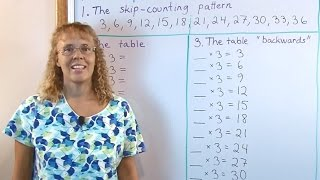By Math Mammoth

This method (I call it a structured drill) allows children to learn the multiplication tables in grade 3 or 4 faster and much more effectively than random drilling!

My book Math Mammoth Multiplication 1 at http://www.mathmammoth.com/multiplica... uses this method also. The book covers both the concept of multiplication and the practice of all the tables. It is available both as an instant download and as printed copy.

See a free example lesson from the book:
http://www.mathmammoth.com/preview/Mu...

In the video, I use the table of 3 as an example and go through this three-step method.

In the first step, children memorize the skip-counting list. For the table of 3 this is: 3, 6, 9, 12, 15, 18, 21, 24, 27, 30, 33, 36. They also practice saying it backwards, from 36 to 3.

In the second step, we work with the table itself, but it is written in order so the child can see its structure. The " randomness " comes from me (the teacher) pointing to various problems in a random order. I also like to point out certain benchmark facts to students: 10 x 3 is 30 and 5 x 3 = 15 are such facts because they're in the 10s table and in the 5s table, which the children probably have learned first.

We also practice the tables " backwards " where the " answers " are written and the student tells how many times 3 they are. For example, I point to ___ x 3 = 24, and the child is supposed to say " 8 " . This type of practice makes children learn basic division facts at the same time without realizing it! This really helps third grade students because division is also a topic for third grade math.# Math Antics - What Is Arithmetic?

##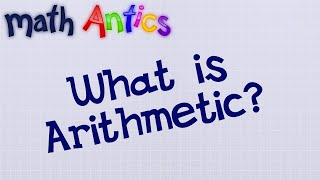By mathantics# 3.OA.6 - Meaning of Division (Singapore Math)

##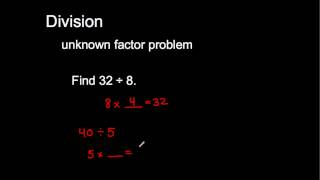By MathwithMrAlmeda

Mr. Almeida explains the meaning of division as an "unknown factor problem." This illustrates 3.OA.6 in the grade 3 Common Core Standards for Mathematics.# Math Antics - Long Division

##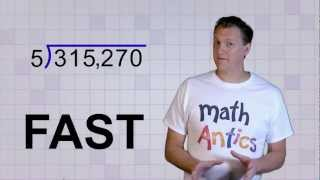By mathantics# Math Antics - Basic Division

##By Lumos Learning# 01 - Learn Order Of Operations (5th Grade Math)

##By Lumos Learning

This is just a few minutes of a complete course. Get all lessons & more subjects at: http://www.MathTutorDVD.com​. This lesson teaches how to use the rules of order of operations to solve problems.# Least Common Multiple LCM

##By tecmath# 08 - Learn Synthetic Division of Polynomials - Part 1

##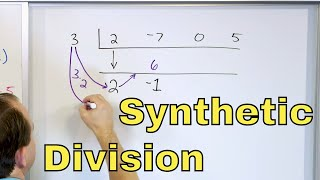By Math and Science

Quality Math And Science Videos that feature step-by-step example problems!# Math Antics - Long Division with 2-Digit Divisors

##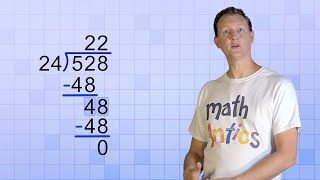By# Math Antics - Integer Multiplication & Division

##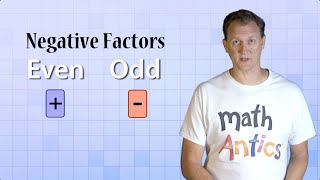By mathantics# Math Antics - Decimal Arithmetic

##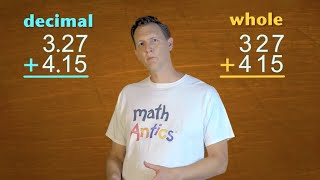By# Master the Order of Operations (PEMDAS) in Math - [5-7-5]

##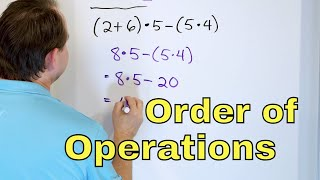By Math and Science

Quality Math And Science Videos that feature step-by-step example problems!# Algebra Basics: What Is Algebra? - Math Antics

##By Lumos Learning

This video gives an overview of Algebra and introduces the concepts of unknown values and variables. It also explains that multiplication is implicit in Algebra.# Learn the Meaning of Dividing Decimals - 

##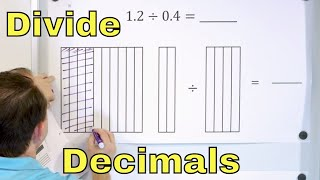By Math and Science# Math Antics - Exponents and Square Roots

##By mathantics# Math Antics - Comparing Fractions

##By mathantics# 05 - Polynomial Long Division - Part 1 (Division of Polynomials Explained)

##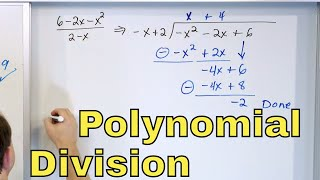By Math and Science

Quality Math And Science Videos that feature step-by-step example problems!# Even and Odd Numbers - Basic Introduction

##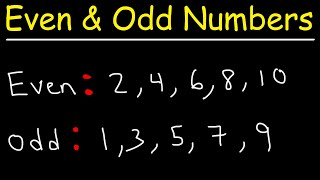By The Organic Chemistry Tutor# Algebra Basics: Laws Of Exponents - Math Antics

##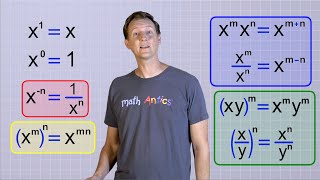By mathantics# 12 - The Factor Theorem, Part 1 (Factoring Polynomials in Algebra)

##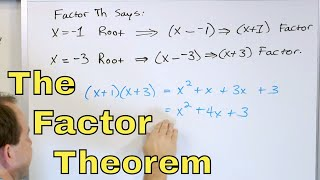By Math and Science

Quality Math And Science Videos that feature step-by-step example problems!